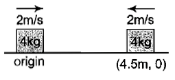A bob of mass m attached to an inextensible string of length l is suspended from verticle support.  The bob rotates in a horizontal circle with an angular speed  about the vertical.  About the point of suspension.

(1) Angular momentum is conserved

(2) Angular momentum changes in magnitude but not in the direction

(3) Angular momentum changes in direction but not in magnitude

(4) Angular momentum changes both in direction and magnitude

High Yielding Test Series + Question Bank - NEET 2020

Difficulty Level:

In free space, a rifle of mass M shoots a bullet of mass m at a stationary block of mass M at a distance D away from it. When the bullet has moved through a distance d towards the block, the centre of mass of the bullet - block system is at a distance of

(1) $\frac{\left(D-d\right)}{M+m}$ from the bullet

(2) $\frac{md+MD}{M+m}$ from the block

(3) $\frac{2md+MD}{M+m}$ from the block

(4) $\frac{\left(D-d\right)M}{M+m}$ from the bullet

Concept Questions :-

Center of mass
High Yielding Test Series + Question Bank - NEET 2020

Difficulty Level:

Blocks A and B are resting on a smooth horizontal surface given equal speeds of 2 m/s in the opposite sense as shown in the figure.At t = 0, the position of blocks are shown, then the coordinates of centre of mass at t = 3s will be

1.  (1, 0)

2.  (3, 0)

3.  (5, 0)

4.  (2.25, 0)

Concept Questions :-

Center of mass
High Yielding Test Series + Question Bank - NEET 2020

Difficulty Level:

A wheel is at rest. Its angular velocity increases uniformly and becomes 80 rad/s after 5 s. The total angular displacement is

Concept Questions :-

Rotation motion : Introduction
High Yielding Test Series + Question Bank - NEET 2020

Difficulty Level:

A force $-\mathrm{F}\stackrel{^}{\mathrm{k}}$ acts on O, the origin of the coordinate system. The torque about the point (1, -1) is :

1. $-\mathrm{F}\left(\stackrel{^}{\mathrm{i}}+\stackrel{^}{\mathrm{j}}\right)$

2. $\mathrm{F}\left(\stackrel{^}{\mathrm{i}}+\stackrel{^}{\mathrm{j}}\right)$

3. $-\mathrm{F}\left(\stackrel{^}{\mathrm{i}}-\stackrel{^}{\mathrm{j}}\right)$

4. $\mathrm{F}\left(\stackrel{^}{\mathrm{i}}-\stackrel{^}{\mathrm{j}}\right)$

Concept Questions :-

Torque
High Yielding Test Series + Question Bank - NEET 2020

Difficulty Level:

Moment of inertia of an object does not depend upon

1.  mass of object

2.  mass distribution

3.  angular velocity

4.  axis of rotation

Concept Questions :-

Moment of inertia
High Yielding Test Series + Question Bank - NEET 2020

Difficulty Level:

A force  is acting on a point . The torque acting about a point  is

1.  0

2.

2.

4.

Concept Questions :-

Torque
High Yielding Test Series + Question Bank - NEET 2020

Difficulty Level:

A thin uniform circular disc of mass M and radius R is rotating in a horizontal plane about an axis passing through its center and perpendicular to its plane with an angular velocity $\omega$. Another disc of the same dimensions but of mass $\frac{1}{4}M$ is placed gently on the first disc co-axially. The angular velocity of the system is

1.  $\frac{2}{3}\mathrm{\omega }$

2.  $\frac{4}{5}\mathrm{\omega }$

3.  $\frac{3}{4}\mathrm{\omega }$

4.  $\frac{1}{3}\mathrm{\omega }$

Concept Questions :-

Angular momentum
High Yielding Test Series + Question Bank - NEET 2020

Difficulty Level:

When a ball of mass = 5kg hits a bat with a velocity = 3 m/s, in positive direction and it moves back with a velocity = 4 m/s, find the impulse in SI units

1. 5

2. 15

3. 25

4. 35

Concept Questions :-

Linear momentum
High Yielding Test Series + Question Bank - NEET 2020

Difficulty Level:

When a torque acting upon a system is zero, then which of the following will be constant

1.  force

2.  Linear momentum

3.  Angular momentum

4.  Linear impulse

Concept Questions :-

Angular momentum